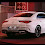## Sunday, August 14, 2022

1.2.Moving the lower left stock from the 0, to the middle to create a 9

Bea Charlover Aca

3.4.you have to move the lower left arrow of the zero to form a nine.

5.you have to move the lower left arrow of the zero to form a nine

6.move the bottom left stick within the 0 to create a 9 and u got 3+6= 9
Char: Duli Leos

7.Tamarizz - Solidera

Move the southwest arrow from the 0 into the middle to make a 9.
So the equation would be 3 + 6 = 9

8.9.3+6=9, move the stick on 0 to turn into a 9

Nick: Iaaaaaaaaaaaa aaaaa Aaaaaaa

10.Zero change one arrow to make nine :)

11.3+6=9

Eve Esece

12.3+6=9

Gold Nightmare

13.3+6=9

Gold Nightmare

14.3+6=9

Shizurie

15.16.3 + 5 = 8

Righo

17.3 + 5 = 8

Righo

18.3 + 5 = 8
Righo

19.Opressora Tym
3+5=8

20.3+6=9

Xafler

21.Name Char: Hinata Hyuga Byakugan

Respuesta: 3 + 5 = 8

22.R= 3+6 = 9

R= 5 + 3 = 8 :)

Samuray de carro ;p

23.3 + 5 = 8

Character Name: Dyaniixz

24.3 + 5 = 8

Character Name: Dyaniixz

25.3+6=9
character name: quatro pesos

26.3+6=9
character name: quatro pesos
i am going to sell this character so can you please change my name to "pablito fiufiu"

27.3+6=9
character name: quatro pesos
i am going to sell this character so can you please change my name to "pablito fiufiu"

28.Move top left side of 0 and turn it into 9. 3+6=9
Kenlux, Antica

29.Bottom left on the zero move it to the middle to turn a 0 into a 9

Esus Ang Warman

30.Psykeeh

3+5 = 8

31.The left bottom of the 0

32.3+6=9, make 9 from 0. Procente

33.Ozud

Move 1 arrow from the 0 as horizontal to make it a 9

34.3+5=8; Lord Neganek

35.3 + 6 = 9
Mian Stone'arrow

36.37.3+6=9, z zera robimy 9

38.hefrus
3+6=9

39.Move the bottom left arrow of the number 0 horizontally in the middle so it makes a number 9 instead. 3+6=9

-Essiee

40.3+5=8
Sir coor

41.also movin one stick from the six, you create a 5 in there, and the spick you move to the 0 creating an 8. So 3+5=8

bea charlover aca

42.3+5=8

Amy Meow

43.Neo
3+5=8 (moving the bottom left vertical arrow from 6 to the center of 0 making them both 5 and 8)
or
3+6=9 (moving the bottom left vertical arrow from 0 to the center making it a 9)

44.This comment has been removed by the author.

45.Move the matchstick (arrow) on the lower left side of the "0" to its center, rotating it to the horizontal position, turning it into a 9.
- Hunter of Dragoes

46.Move the arrow from the 6 to the 0 to make 3+5=8

Xarkost Eilia

47.3+5=8
Knish Icearrow
Ardera

48.Garoto do Sorriso (Yonabra)
3+5=8

49.Bahtats
3+5=8

50.Respuesta: quitándole la flecha de abajo del número 6 la está en la parte lateral del lado izquierdo y se le coloca en el medio del 0
Quedaría 3 + 5 = 8

51.3+6=9 by moving one arrow on the 0 to make it a 9

- Napom

52.Nick: Eriica Sar

https://imgur.com/hgGM1Ia

3+6=9

53.Meregirl
3+5 = 8
https://imgur.com/a/j3gkY8N

54.Lyh

https://imgur.com/a/4gGiBZW

3 + 6 = 9

55.Grove Max, You have to move the arrow from 6 so that there is a 5 and put them after = in the middle of 0 to get 8.

56.Presckot

https://imgur.com/a/tkJsdOm
3 + 5 = 8

57.3 + 6 = 9 - Hard dyal

58.3+0=0

59.